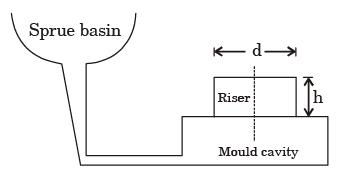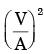Courses

# Past Year Questions: Solidification and Cooling Mechanical Engineering Notes | EduRev

## Mechanical Engineering : Past Year Questions: Solidification and Cooling Mechanical Engineering Notes | EduRev

The document Past Year Questions: Solidification and Cooling Mechanical Engineering Notes | EduRev is a part of the Mechanical Engineering Course Manufacturing and Industrial Engineering.
All you need of Mechanical Engineering at this link: Mechanical Engineering

Question 1:The contraction allowance provided on the pattern and core boxes compensates for the following type of contraction

[ME 1988]

Question 2:Chills are used in moulds to

[ME 1989]

Question 3:Two cubical castings of the same metal and size of 2 cm side and 4 cm side are moulded in green sand. If the smaller casting solidifies in 2 mins, the expected time of solidifications of large casting will be

[ME 1989]

Question 4:When there is no room temperature change, the total shrinkage allowance on a pattern is independent of

[ME 1991]

Question 5:With a solidification factor of 0.97 × 106 s/m2, the solidification time (in seconds) for a spherical casting of 200 mm diameter is

[ME 2003]

Question 6:Volume of a cube of side l and volume of a sphere of radius r are equal. Both the cube and the sphere are solid and of same material. They are being east. The ratio of the solidification time of the cube to the same of sphere is

[ME 2007]

Question 7:A cubic casting of 50 mm side under goes volumetric solidification shrinkage and volumetric solid contraction of 4% and 6% respectively. No riser is used. Assume uniform cooling in all directions. The side of the cube after solidification and contraction is

[ME 2011]

Question 8:A cube shaped casting solidifies in 5 min. The solidification time in min for a cube of the same material, which is 8 times heavier than the original casting, will be

[ME 2013]

Question 9:A cylindrical blind riser with diameter d and height h, is placed on the top of the mold cavity of a closed type sand mould as shown in the figure. If the riser is of constant volume, then the rate of solidification in the riser is the least when the ratio h : d is[ME 2014,Set-3]

Question 10:The solidification time of a casting is proportional towhere V is the volume of the casting and A is the total casting surface area losing heat. Two cubes of same material and size are cast using sand casting process. The top face of one of the cubes is completely insulated. The ratio of the solidification time for the cube with top face insulated to that of the other cube is

[ME 2015,Set-1]

Question 11:28. Equal amounts of a liquid metal at the same temperature are poured into three moulds made of steel, copper and aluminum. The shape of the cavity is a cylinder with 15 mm diameter. The size of the moulds are such that the outside temperature of the moulds do not increase appreciably beyond the atmospheric temperature during solidification. The sequence of solidification in the mould from the fastest to slowest is (Thermal conductivities of steel, copper and aluminum are 60.5, 401 and 237 W/mK, respectively. Specific heats of steel, copper and aluminum are 434, 385 and 903 J/ kgK, respectively. Densities of steel, copper and aluminum are 7854, 8933 and 2700 kg/m3, respectively.)

[ME 2016,Set-3]

Question 12:A cast Steel slab of dimension 30 × 20 × 5 cm is poured horizontally using a side riser. The riser is cylindrical in shape with diameter and height, both equal to D. The freezing ratio of the mould is

[PI 2005]

Question 13:A solid cylinder of diameter D and height equal to D, and a solid cube of side L are being sand cast by using the same material. Assuming there is no superheat in both the cases, the ratio of solidification times of the cylinder to the solidification time of the cube is

[PI 2009]

Offer running on EduRev: Apply code STAYHOME200 to get INR 200 off on our premium plan EduRev Infinity!

## Manufacturing and Industrial Engineering

91 videos|52 docs|59 tests

,

,

,

,

,

,

,

,

,

,

,

,

,

,

,

,

,

,

,

,

,

;